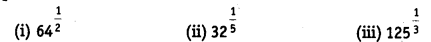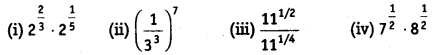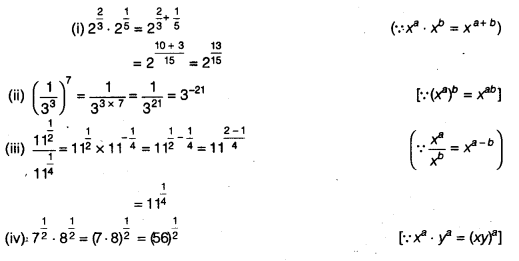NCERT Solutions for Class 9 Maths Chapter 1 Number Systems Ex 1.6 are part of NCERT Solutions for Class 9 Maths. Here we have given NCERT Solutions for Class 9 Maths Chapter 1 Number Systems Ex 1.6.

 Board CBSE Textbook NCERT Class Class 9 Subject Maths Chapter Chapter 1 Chapter Name Number Systems Exercise Ex 1.6 Number of Questions Solved 3 Category NCERT Solutions

## NCERT Solutions for Class 9 Maths Chapter 1 Number Systems Ex 1.6

Question 1.
Find:Solution:Question 2.
Find:Solution:Question 3.
Simplify:Solution:We hope the NCERT Solutions for Class 9 Maths Chapter 1 Number Systems Ex 1.6, help you. If you have any query regarding NCERT Solutions for Class 9 Maths Chapter 1 Number Systems Ex 1.6, drop a comment below and we will get back to you at the earliest.